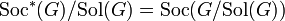# Babai-Beals filtration

## Definition

Let$G$ be a finite group. The Babai-Beals filtration of$G$ is the following ascending chain of subgroups of$G$:$1 \le \operatorname{Sol}(G) \le \operatorname{Soc}^*(G) \le \operatorname{PKer}(G) \le G$

where:

•$1$ denotes the trivial subgroup of$G$.
•$\operatorname{Sol}(G)$ denotes the solvable radical of$G$.
•$\operatorname{Soc}^*(G)$ denotes the socle over solvable radical of$G$, i.e.,$\operatorname{Soc}^*(G)/\operatorname{Sol}(G) = \operatorname{Soc}(G/\operatorname{Sol}(G))$.
•$\operatorname{PKer}(G)$ denotes the permutation kernel of$G$.

Here is what we can say about the successive quotients:

•$\operatorname{Sol}(G)$ is a solvable group.
•$\operatorname{Soc}^*(G)/\operatorname{Sol}(G)$ is a direct product of simple non-abelian groups (with some of them possibly isomorphic).
•$\operatorname{PKer}(G)/\operatorname{Soc}^*(G)$ is isomorphic to a subgroup of the external direct product of the outer automorphism groups of some simple non-abelian groups (the same ones that appear in the quotient$\operatorname{Soc}^*(G)/\operatorname{Sol}(G)$, with the same repetitions). Hence, by Schreier's conjecture (which has been proved conditional to the classification of finite simple groups) it is a solvable group.
•$G/\operatorname{PKer}(G)$ has order at most$\log_{60}|G|$, hence is relatively small compared to the size of$G$.